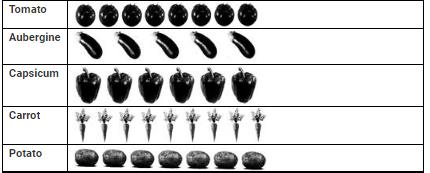Courses

# Test: Data Handling - 3

## 20 Questions MCQ Test Mathematics (Maths) for Class 3 | Test: Data Handling - 3

Description
This mock test of Test: Data Handling - 3 for Class 3 helps you for every Class 3 entrance exam. This contains 20 Multiple Choice Questions for Class 3 Test: Data Handling - 3 (mcq) to study with solutions a complete question bank. The solved questions answers in this Test: Data Handling - 3 quiz give you a good mix of easy questions and tough questions. Class 3 students definitely take this Test: Data Handling - 3 exercise for a better result in the exam. You can find other Test: Data Handling - 3 extra questions, long questions & short questions for Class 3 on EduRev as well by searching above.
QUESTION: 1

### DIRECTION: The given pictograph shows the number of 4 different kinds of plants Mohit bought. Study the pictograph and answer the questions.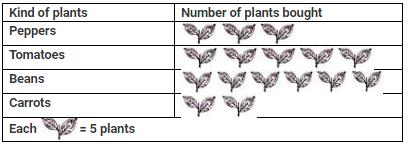Q. What is the total number of plants he buy?

Solution:

Number of pepper plants he bought = 3×5=15
Number of tomato plants he bought = 5×5=25
Number of bean plants he bought = 6×5=30
Number of carrot plants he bought = 2×5=10
∴Total number of plants Mohit bought = 15+25+30+10 =80

QUESTION: 2

### DIRECTION: The given pictograph shows the number of children who came to story hour each week. Study the pictograph and answer the following questions.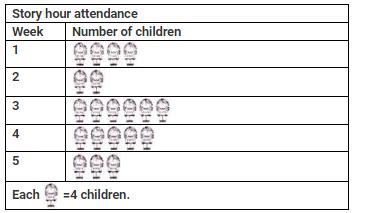Q. How many children came to story hour in week 4?

Solution:

Number of children came in week 4 = 5 × 4 = 20

QUESTION: 3

### DIRECTION: The given pictograph shows the number of children who came to story hour each week. Study the pictograph and answer the following questions.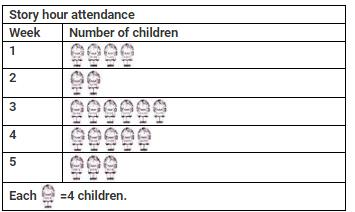Q. How many more children came for story hour in week 3 than in week 2?

Solution:

Number of children came in week 3 = 6 × 4 = 24
Number of children came in week 2 = 2 × 4 = 8
∴ Difference = 24 − 8 = 16 So, 16 more children came for story hour in week 3 than in week 2.

QUESTION: 4

DIRECTION: The given pictograph shows the number of children who came to story hour each week. Study the pictograph and answer the following questions.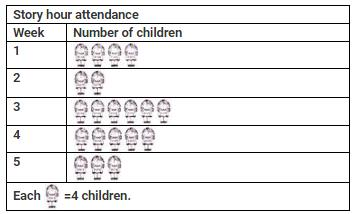Q. Total number of children came for story hours in all weeks is _______.

Solution:

Number of children came in week 1 = 4×4=16
Number of children came in week 2 = 2×4=8
Number of children came in week 3 = 6×4=24
Number of children came in week 4 =5×4=20
Number of children came in week 5 =3×4=12
∴Total number of children came for story hour in all weeks =16+8+24+20+12=80

QUESTION: 5

DIRECTION: The given pictograph shows the number of children who came to story hour each week. Study the pictograph and answer the following questions.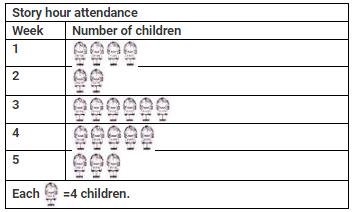Q. How many more children came to story hour in week 1 and week 5 together than in week 3?

Solution:

Number of children came in week 3 = 24
Number of children came in week 1 and week 5 together =16+12=28 So, number of more children who came to story hour in week 1 and week 5 together than in week 3=28−24=4

QUESTION: 6

Study the given table and answer the question based on the data in the table.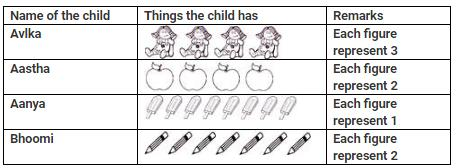Q. Who had the maximum number of items?

Solution: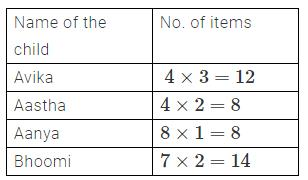∴ Bhoomi has the maximum number of items.

QUESTION: 7

The given pictograph graph shows the number of bookmarks each student made. Study the pictograph and answer the following question.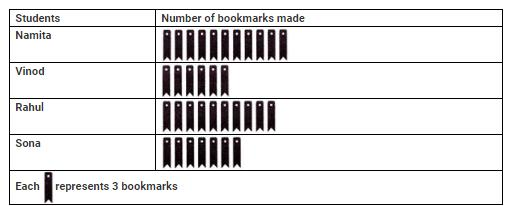Q. How many less bookmarks did Namita make than Sona and Vinod together?

Solution:

Number of bookmarks made by Sona =7×3=21
Number of bookmarks made by Vinod = 6×3=18
∴ Number of bookmarks made by Sona and Vinod together =21+18=39
Now, number of bookmarks made by Namita =11×3=33
∴ Required difference =39−33=6. So, Namita made 6 less bookmarks than Sona and Vinod together.

QUESTION: 8

The given graph shows the number of books read by pupils in a class from July to December. Study the given graph and answer the following question.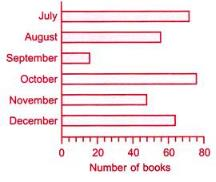Q. How many books were read altogether from July to November?

Solution:

Number of books read in July = 72
Number of books read in August = 56
Number of books read in September =16
Number of books read in October = 76
Number of books read in November = 48
∴Total number of books read from July to November =72+56+16+76+48=268

QUESTION: 9

The given pictograph shows the number of sweaters ordered of different size. Study the pictograph and answer the following question.Q. Which size of exactly 20 sweaters were ordered?

Solution:

Number of large size sweaters ordered = 4 × 5 = 20
∴ large size sweaters were ordered exactly 20.

QUESTION: 10

What is the total number of cellphones in the given figure: [Here 1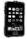= 100 cellphones]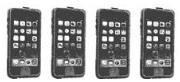Solution:
QUESTION: 11

Direction: A survey was conducted on a group of 90 students. The information obtained is shown below: [Here 1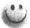= 10 persons]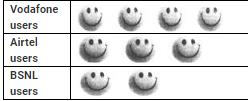Q. The number of vodafone users is ______

Solution:
QUESTION: 12

Direction: A survey was conducted on a group of 90 students. The information obtained is shown below: [Here 1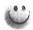= 10 persons]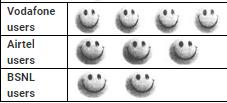Q. The number of Airtel users is _________

Solution:
QUESTION: 13

Direction: A survey was conducted on a group of 90 students. The information obtained is shown below: [Here 1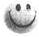= 10 persons]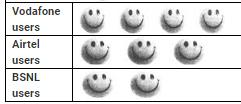Q. The number of BSNL users is __________

Solution:
QUESTION: 14

If each of the following balls is arranged in a group of 5 balls, how many such groups are possible?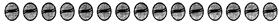Solution:
QUESTION: 15

Find the total number of tigers in the given figure? [Here 1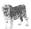= 6 tigers]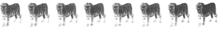Solution:
QUESTION: 16

If * represents 12 pens then which one of the following will represents 72 pens?

Solution:
QUESTION: 17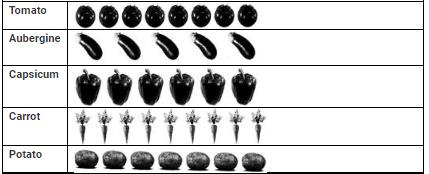Q. How many capsicums are there?

Solution:
QUESTION: 18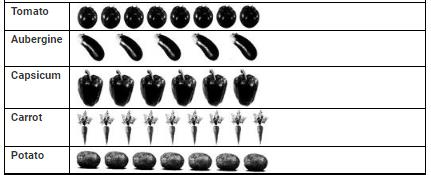Q. Which kind of vegetable is minimum in number?

Solution:
QUESTION: 19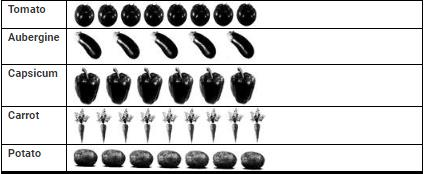Q. Which kind of vegetable is maximum in number?

Solution:
QUESTION: 20